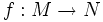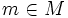# Immersion

## Definition

Let$M$ and$N$ be differential manifolds. A differentiable map$f:M \to N$ is termed an immersion at a point$m \in M$, the induced map$df_m: T_mM \to T_{F(m)}N$ is injective.$f$ is called an immersion if it is an immersion at every point in$M$.

We also say that$M$ is an immersed manifold in$N$.